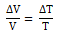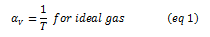It contracts on heating between 0 °C and 4 °C. The volume of a given amount of water decreases as it is cooled from room temperature until its temperature reaches 4 °C, [Fig.(a)]. Below 4 °C, the volume increases, and therefore the density decreases [Fig. (b)].

This means that water has a maximum density at 4 °C. This property has an important environmental effect:Gases at ordinary temperature expand more than solids and liquids. For liquids, the coefficient of volume expansion is relatively independent of the temperature. However, for gases, it is dependent on temperature. For an ideal gas, the coefficient of volume expansion at constant pressure can be found from the ideal gas equation:

PV = μ R T

At constant pressure

P∆V = μ R ∆Ti.e.At 0 °C, αv = 3.7 × 10–3 K–1, which is much larger than that for solids and liquids.

There is a simple relation between the coefficient of volume expansion (αV) and coefficient of linear expansion (αl).